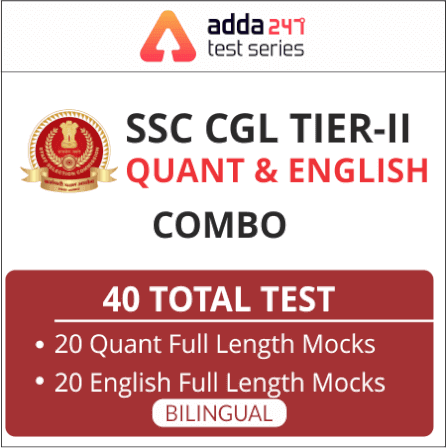Home Maths Notes Study Notes & Questions for SSC Exams: SI & CI

# Study Notes & Questions for SSC Exams: SI & CI## Important Notes On SI & CI For SSC Exams :

Quantitative Aptitude is an equally important section for SSC CGLSSC CHSL, SSC MTS exams and has even more abundant importance in some other exams conducted by SSC. Generally, there are questions asked related to basic concepts and formulas of Ratio & Proportion.

To let you make the most of QUANT section, we are providing important facts related to Ratio & Proprtion. Also, RRB NTPC 2019 Exam is nearby with bunches of posts for the interested candidates in which quantitative aptitude is a major part. We have covered important notes and questions focusing on these prestigious exams. We wish you all the best of luck to come over the fear of Mathematics section.

Simple Interest & Compound Interest

Simple Interest1. If a certain sum in T years at R% per annum amounts to Rs. A, then the sum will be

2. The annual payment that will discharge a debt of Rs. A due in T years at R% per annum is .Annual payment=#### Click Here to Attempt More Questions From Quantitative Aptitude For SSC CGL

3. If a certain sum is invested in n types of investments in such a manner that equal amount is obtained on each investment where interest rates are R₁, R₂, R₃ ……, R_n, respectively and time periods are T₁, T₂, T₃, ……, T_n, respectively, then the ratio in which the amounts are invested is

4. If a certain sum of money becomes n times itself in T years at simple interest, then the rate of interest per annum is

5. If a certain sum of money becomes n times itself at R% per annum simple interest in T years, then

6. If a certain sum of money becomes n times itself in T years at a simple interest, then the time T in which it will become m times itself is given by

#### Click Here For Study Notes on Quantitative Aptitude For SSC CGL

7. Effect of change of P, R and T on simple interest is given by the following formula:

8. If a certain sum of money P lent out at SI amounts to A₁ in T₁ years and to A₂ in T₂ years, then

9. If a certain sum of money P lent out for a certain time T amounts to A₁ at R₁ % per annum and to A₂ at R₂ % per annum, then

10. If an amount P₁ lent at the simple interest rate of R₁ % per annum and another amount P₂ at the simple interest rate of R₂ % per annum, then the rate of interest for the whole sum is

Compound Interest

1. (a) The amount A due after t years, when a principal P is given on compound interest at the rate R% per annum is given by

2. If the interest is compounded half-yearly, then

3. If the interest is compounded quarterly, then

4. When the rates of interest are different for different years, say R₁, R₂, R₃ percent for first, second and third year, respectively, then

5. When the time is given in the form of fraction, say 23/4 years, then,

6. (a) The difference between the compound interest and the simple interest on a certain sum of money for 2 years at R% per annum is given by

(b) The difference between the compound interest and the simple interest on a certain sum of money for 2 years at R% per annum is given by

7. If a certain sum becomes n times in t years at compound interest, then the same sum becomes n^m times in mt years.

8. If a certain sum becomes n times in t years, then the rate of compound interest is given by

9. If a certain sum of money at compound interest amounts to Rs. x in A years and to Rs. y in B years, then the rate of interest per annum is

10. If a loan of Rs. P at R% compound interest per annum is to be repaid in n equal yearly instalments, then the value of each instalment is given by

You May Also Like To Read :